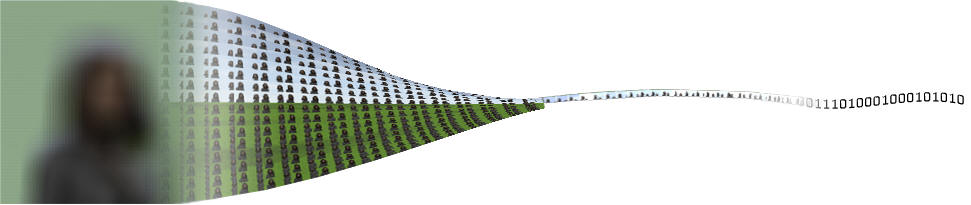Pseudo Video Sequence Coding of Integral Imaging based 3D Images

There is a significant amount of inherent redundancy in an integral image. Applying a 2D-image coding scheme to an integral image fail to extract and reduce this redundancy efficiently. We have proposed a pre-processing and compression scheme that aims to enhance the compression efficiency of integral images. The scheme first transforms the still integral image into a pseudo video sequence consisting of sub-images, which is then compressed using an H.264 video encoder. Thereby leveraging on the efficient temporal and spatial coding tools of the H.264-standard for coding integral images.
The improvement in compression efficiency of using this scheme is evaluated and presented. Different parameterizations are also investigated, which affects the coding efficiency at low range bitrates. An average PSNR increase of 5.7 dB or more, compared to JPEG 2000, is observed on a set of reference images. Compared to other coding schemes the introduced artifacts are distributed more homogenously within the depicted 3D-volume.

Proposed coding scheme

To lay the groundwork we first define an integral imaging based 3D-image (II-frame) with a resolution of M x N pixels as

II(m, n) = [IIR,  IIG,  IIB]T,          (1)

where m = 0, 1, ..., M-1 and n = 0, 1, ... N-1 are the horizontal and vertical position of a II-frame pixel, which constitute a RGB-triplet. In our simulations we assume a lens array with rectangular lenslets, but the proposed scheme is applicable to hexagonal and lenticular lenslets as well provided that the number of views are sufficiently high. This gives K·L elementary images (EI) with a resolution of U x V pixels that are defined as

EIk,l (u, v) = II(k·U + u, l·V + v),    (2)

where k = 0, 1, ..., K-1 and l = 0, 1, ... L-1 is the position of each EI and u= 0, 1, ..., U-1 and v = 0, 1, ... V-1 is the pixel positions within every EI.

As a first step in our proposed coding scheme we extract the set of sub-images (SI) according to

SIu,v( k, l) = II(k·U + u, l·V + v).    (3)

where consequently each of the U·V SIs have a resolution of K x L pixels. Figure 1 shows examples of the above defined images II, EI and SI.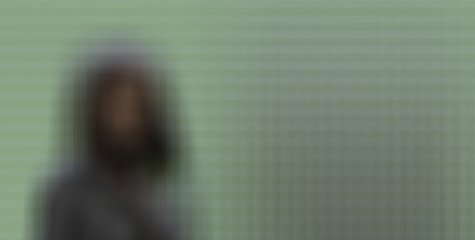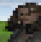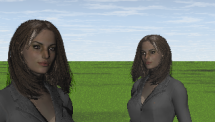(a) II-frame (b) EI (c) SI (d) 2D-image

Figure 1. Synthesized II-frame divided into its component parts. (d)  shows the scene captured by a 2D-camera merely for reference.

The SIs are then considered as pictures in a pseudo video sequence (PVS) according to

PV S(t) = SIu(t),v(t)(k, l) = II(k·U + u(t), l·V + v(t)),     (4)

where t is the pseudo time index, or picture number, of the PVS. Selecting different functions u(t) and v(t), or selection orders, results in PVSs with different coding properties. The PVS is then coded using a H.264/MPEG-4 AVC encoder. The three steps are illustrated in Figure 2.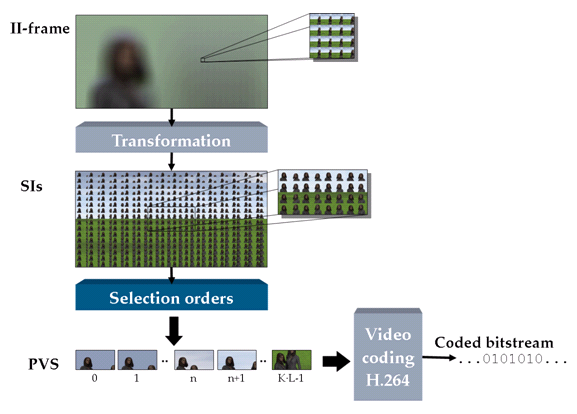Figure 2.  Block diagram of the proposed coding scheme

Results

The proposed coding scheme outperforms JPEG2000 and other proposed PVS-schemes for resolution prioritizing II-techniques where the number of lenslets is large. For depth prioritizing II, with a lower number of high resolution EI, the benefits are less. A proper choice of selection order for the proposed scheme becomes more important at low bit rates. Compared to the references the proposed coding scheme distributes the coding artifacts homogenously over the 3D-scene's depth.

Proposed scheme vs. 2D-image coding and other PVS-based coding schemes ("Car")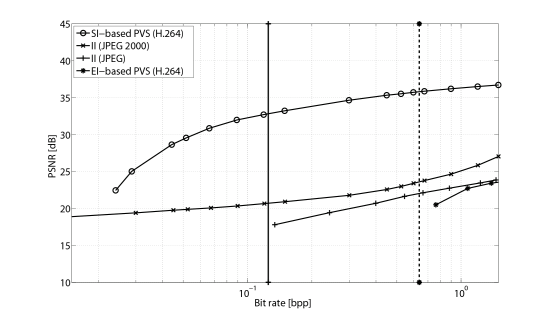Different selection orders' effect on coding efficiency ("Twins")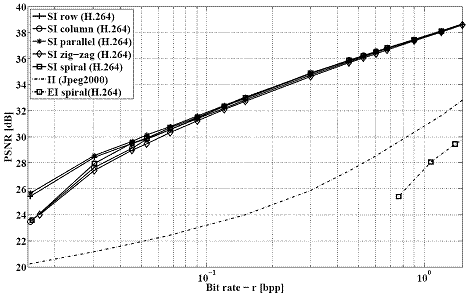Lens array properties effect on coding efficiency ("Cuboid")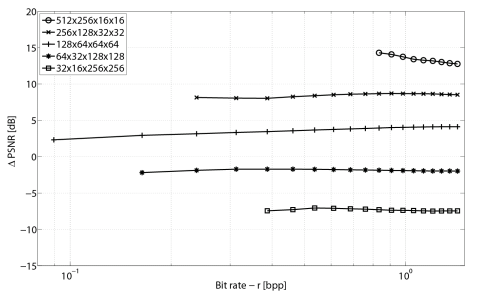Induced coding artifacts ("Apples", front view @ 0.15 bpp)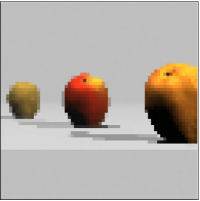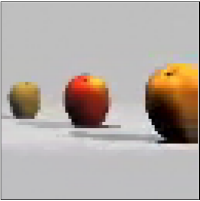Original (24 bpp)                  Proposed SI-based PVS coding scheme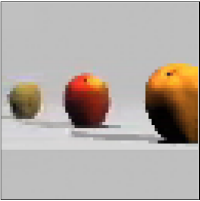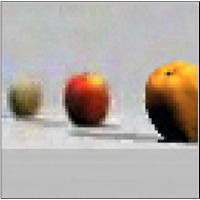EI-based PVC coding scheme                           JPEG2000

Publications

"Multiview image coding scheme transformations: artifact characteristics and effects on perceived 3D quality"
Roger Olsson and Mårten Sjöström
Proceedings of Stereoscopic Displays and Applications, January 2010
Web page accompanying paper

"Empirical Rate-Distortion Analysis of JPEG2000 3D and H.264/AVC Coded Integral Imaging Based 3D-Images"
Roger Olsson
Proceedings of 3DTV Conference, IEEE/EURASIP/MPEG-IF, May 2008
Web page accompanying paper

"A novel quality metric for evaluating depth distribution of artifacts in coded still 3D images",
Roger Olsson and Mårten Sjöström
Electronic Imaging - Stereoscopic Displays and Applications, IS&T/SPIE, January 2008

"A depth dependent quality metric for evaluation of coded integral imaging based 3D-images",
Roger Olsson
and Mårten Sjöström
Proccedings of 3DTV-Conference, IEEE, May 2007

"Evaluation of Combined Pre-Processing and H.264-Compression Schemes for 3D Integral Images",
Roger Olsson
, Mårten Sjöström  and Youzhi Xu,
Proceedings of Electronic Imaging - VCIP,
SPIEIS&T/SPIE, January 2007

"A Combined Pre-processing and H.264-compression Scheme for 3D Integral Images"
Roger Olsson, Mårten Sjöström and Youzhi Xu,
Proceedings of ICIP, IEEE, October 2006